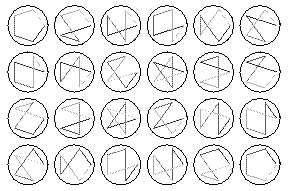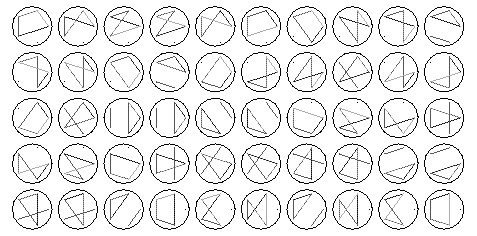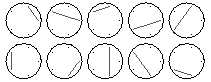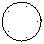# Stirling numbers of the first kind

The Stirling numbers of the first kind $s(n, k)$ count the number of ways to permute a list of $n$ items into $k$ cycles.

For example,
the list {1, 2, 3, 4} can be permuted into two cycles in the followingways:

• {{1,3,2},{4}}
• {{1,2,3},{4}}
• {{1,4,2},{3}}
• {{1,4,2},{3}}
• {{1,2,4},{3}}
• {{1,2},{3,4}}
• {{1,4,3},{2}}
• {{1,3,4},{2}}
• {{1,3},{2,4}}
• {{1,4},{2,3}}
• {{1},{2,4,3}}
• {{1},{2,3,4}}
There are 11 such permutations, thus s(4, 2) = 11.

Here are some illegible diagrams showing the cycles for permutations of a list
with five elements.

s(5, 1) = 24:s(5, 2) = 50:s(5, 3) = 35:s(5, 4) = 10:s(5, 5) = 1: